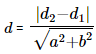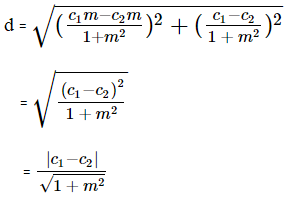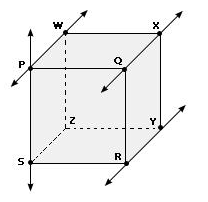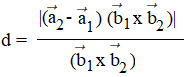# Shortest Distance between Two Lines

The shortest distance between two parallel lines is equal to determining how far apart lines are. This can be done by measuring the length of a line that is perpendicular to both of them. We may derive a formula using this approach and use this formula directly to find the shortest distance between two parallel lines. For two non-intersecting lines lying in the same plane, the shortest distance is the distance that is shortest of all the distances between two points lying on both lines. In this page, we will study the shortest distance between two lines in detail.

Table of Content:

## Distance between two Straight Lines

The distance between two straight lines in a plane is the minimum distance between any two points lying on the lines. In geometry, we often deal with different sets of lines such as parallel lines, intersecting lines or skew lines.

 Distance between Two Parallel Lines The distance is the perpendicular distance from any point on one line to the other line. Distance between Two Intersecting Lines The shortest distance between such lines is eventually zero. Distance between Two Skew Lines The distance is equal to the length of the perpendicular between the lines.

Related Articles:

Straight Lines

3D Geometry

Distance Formula

## Shortest Distance Between Two Parallel Lines

Formula to find distance between two parallel line:

Consider two parallel lines are represented in the following form :

y = mx + c1 …(i)

y = mx + c2 ….(ii)

Where m = slope of line

Then, the formula for shortest distance can be written as under :

d = $\frac{|c_2 – c_1|}{\sqrt{1+m^2}}$

If the equations of two parallel lines are expressed in the following way :

ax + by + d1 = 0

ax + by + d2 = 0

then there is a little change in the formula.Remark: The perpendicular distance between parallel lines is always a constant, so we can pick any point to measure the distance.

### Proof

First calculate the difference of two intercepts of above lines, (i) and (ii), through the perpendicular line given by

Here -y = -x/m and -1/m is the slope of perpendicular line.

We have two lines, y = mx + c1 and y = -x/m

Now, mx + c1 = -x/m

x = -c1m/1+m2

Again, y = c1/1+m2

So, (-c1m/1+m2, c1/1+m2) is the intersecting point of the perpendicular line and first line.

Similarly, solving for second equation, the intersecting point of perpendicular line and second line is (-c2m/1+m2, c2/1+m2)

Distance between these two points:In vector Form:

If $\vec{r}=\vec{a_1} + \lambda \vec{b}$ and $\vec{r}=\vec{a_2} + \mu \vec{b}$

Then,

d = $|\frac{\vec{b} \times (\vec{a_2}-\vec{a_1})}{|\vec{b}|}|$

## Shortest Distance Between Skew Lines

A set of lines which do not intersect each other any point and are not parallel are called skew lines (also known as agonic lines). Such set of lines mostly exist in three or more dimensions.

For Example: In below diagram, RY and PS are skew lines among the given pairs.Distance formula:

The distance between two lines of the form, l1 = a1 + t b1 and l2 = a2 + t b2## Solved Examples

Example 1: Find the distance between two parallel lines y = x + 6 and y = x – 2.

Solution: Given equations are of the form, y = mx + c

Here, m = 1, c1 = 6, c2 = -2

Formula: d = |c1 – c2|/√(1 + m2)

Therefore, d = 8/√2 or 5.65 Units.

Example 2: Find the shortest distance between lines

$\vec{r}$ = i + 2j + k + $\lambda$( 2i + j + 2k) and $\vec{r}$ = 2i – j – k + $\mu$( 2i + j + 2k)

Solution:

Using formula, d = $|\frac{\vec{b} \times (\vec{a_2}-\vec{a_1})}{|\vec{b}|}|$

Here, $|\vec{b} \times (\vec{a_2}-\vec{a_1})|$$\begin{vmatrix} i & j & k\\ 2 & 1 &2 \\ 1 & -3 &-2 \end{vmatrix}$

= |4i – 6j – 7k|

= √101

And $|\vec{b}|$ = 3

Therefore, d = √101/3